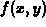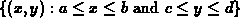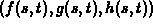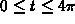Next: Saving and printing Up: Getting Started With Previous: Plotting Functions.

## Three dimensional plotting in Maple.

Maple can plot surfaces in three dimensions. The command syntax to plot a functionover the domainis

```  > plot3d(f(x,y),x=a..b,y=c..d);
```

There are optional arguments you can supply to modify default plotting parameter values; execute ?plot3d to learn more. Fortunately, most of these options can be invoked interactively from the menus in the plot3d window.

It is also possible to plot surfaces given by equations of the form, with a command like the following.

```  > plot3d([f(s,t),g(s,t),h(s,t)],s=a..b,y=c..d);
```

The first time you use the plot3d command, you will notice that there are quite a few menu items you can use to change the appearance of the plot. Note that your changes do not take effect until you click the middle mouse button (MB2) in the window. It is also easy to change your viewpoint of the surface. Simply click MB1 anywhere on the plot. A box should appear that you can move around by dragging with MB1. Once you are done moving the box around, click the middle mouse button (MB2) in the window and the surface will be redrawn.

Maple can also plot curves in three dimensions, but not with the plot3d command. Instead, there is a package of special-purpose plotting routines that you can access that includes a command for plotting parametric curves in three dimensions. To load this package, you must execute the command

```  > with(plots);
```

This makes all of the commands in the package plots available to you. To get a listing of these commands, type ?plots. The particular command we are interested in is spacecurve. To find out more about the spacecurve command, type

```  > ?plots,spacecurve
```

A simple call to spacecurve has the following form.

```  > spacecurve([f(t),g(t),h(t)],t=a..b);
```

whereare functions or expressions. For example, the command

```  > spacecurve([cos(t),sin(t),t],t=0..4*Pi);
```

plots the spiral curvefor. Note that the help for spacecurve says you can also plot more than one curve at the same time, but this feature does not seem to work.Next: Saving and printing Up: Getting Started With Previous: Plotting Functions.

William W. Farr
Mon Aug 28 09:31:56 EDT 1995